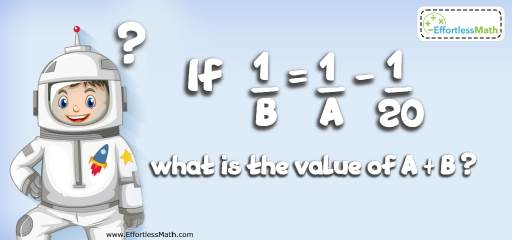# Intelligent Math Puzzle – Challenge 92

Some Math puzzles like the following one stumped even the smartest people. Can You Solve It? The solution is also given.## Challenge:

If A and B are integers and $$\frac{1}{B}=\frac{1}{A}-\frac{1}{20}$$ what is the value of A+B?

### The Absolute Best Book to challenge your Smart Student!

$$\frac{1}{B} =\frac{1}{A} -\frac{ 1}{20}$$
Therefore, A is less than B. Why?
Plug in different values for A.
Let’s begin with 2. If A $$= 2 → \frac{1}{B} = \frac{ 1}{2} – \frac{ 1}{20} → \frac{ 1}{B} = \frac{ 9}{20} → 9B = 20$$
B is an integer. Therefore, B cannot be $$\frac{ 20}{9}$$
A $$= 3 → \frac{ 1}{B} = \frac{ 1}{3} – \frac{ 1}{20} → \frac{ 1}{B} = \frac{ 17}{60} → 17B = 60$$
A $$= 4 → \frac{ 1}{B} = \frac{ 1}{4} – \frac{ 1}{20} → \frac{ 1}{B} = \frac{4}{20} → 4B = 20 → B = 5$$ Bingo!
A = 4 and B = 5 → A + B = 9

The Best Books to Ace Algebra

### What people say about "Intelligent Math Puzzle – Challenge 92 - Effortless Math: We Help Students Learn to LOVE Mathematics"?

No one replied yet.

X
45% OFF

Limited time only!

Save Over 45%

SAVE $40 It was$89.99 now it is \$49.99